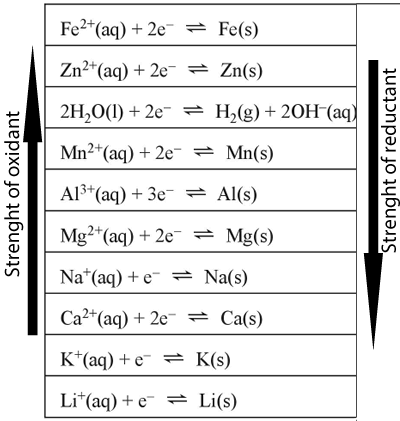Electrical energy Oxidising and reducing strengths.For each of the situations below write the oxidation, reduction and overall reactions. 1) Aluminium nitrate is added to a solution of potassium nitrate and a solid piece of magnesium added. Solution 2) Calcium metal is placed in a solution of aluminium nitrate and magnesium nitrate. Solution 3) Sodium metal is placed in a solution of aluminium nitrate. Solution 4) Iron(II) nitrate and zinc nitrate solutions are mixed and a piece of aluminium metal placed in the resulting solution. Solution 5) A piece of aluminium metal is placed in a iron(II) sulfate solution. a) Identify the oxidant and the reductant b) Write the oxidation and reduction reactions. c) Write the overall reaction occuring in the solution. Solution 6) A student has four solutions and a piece of magnesium metal. The solutions are : iron(II)nitrate, sodium nitrate, calcium nitrate and potassium nitrate. The student dipped the aluminium in each solution. a) In which solution was there a metal deposited on the magnesium? b) When the student dipped the magnesium in the calcium nitrate solution a reaction took place. Write an overall equation for the reaction that occured. c) The student then mixed together the solutions of calcium nitrate and potassium nitrate and placed the magnesium in the resulting solution. She noticed a reaction taking place. Write the overall equation for the reaction taking place. Solution

Home